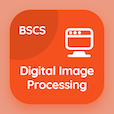Online CS Degree Courses

Database Systems MCQs

Database Systems MCQ - Topics

Learn Notations for Modeling Data Multiple Choice Questions (MCQ Quiz), Notations for Modeling Data quiz answers PDF to learn database systems course for database systems online classes. Database Design and ER Model Multiple Choice Questions and Answers (MCQs), Notations for Modeling Data quiz questions for master's degree in computer science. Notations for Modeling Data Book PDF: constraints in dbms, entity relationship diagrams, specialization test prep for online computer engineering programs.

"Binary relationship sets in UML can be represented by just drawing a line connecting" MCQ PDF: notations for modeling data App APK with entity sets, relationships, attributes, and objects choices for master's degree in computer science. Study notations for modeling data quiz questions for merit scholarship test and certificate programs for computer information science.

## MCQ on Notations for Modeling Data Quiz

MCQ: Binary relationship sets in UML can be represented by just drawing a line connecting

Entity sets
Relationships
Attributes
Objects

MCQ: UML stands for

Unified Monitoring Language
Unified Modeling Language
Uniform Modeling Language
Uniform Monitoring Language

MCQ: A class diagram is similar to an

E-R diagram
Use case diagram
Activity diagram
Implementation diagram

MCQ: UML diagrams that shows the system components along with their interconnections, is known as

Class diagram
Use case diagram
Implementation diagram
Activity diagram

MCQ: The most widely used notations for modeling data, is

XML
UML
E-R diagram
Both A and B

### More Quizzes on Database Systems Book